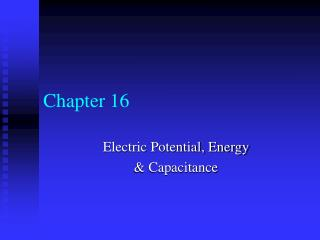Download PresentationChapter 16Chapter 16 - PowerPoint PPT Presentation

Download PresentationChapter 16
An Image/Link below is provided (as is) to download presentation

Download Policy: Content on the Website is provided to you AS IS for your information and personal use and may not be sold / licensed / shared on other websites without getting consent from its author. While downloading, if for some reason you are not able to download a presentation, the publisher may have deleted the file from their server.

- - - - - - - - - - - - - - - - - - - - - - - - - - - E N D - - - - - - - - - - - - - - - - - - - - - - - - - - -
Presentation Transcript

1. Chapter 16 Electric Potential, Energy & Capacitance

2. Electric potential • Gravitational Potential Energy • 3 masses at the same height • Different Potential Energies, PE = mgh • Same PE per unit mass • Electric Potential—PE per unit charge • Called “voltage” • Units of Volts (V)

3. Electric potential • Back to gravity • PE is only measurable between two separate points • PE at point A is meaningless without knowing how far away point B is • h = height above the ground • Ground can be the 1st floor, 2nd floor, 3rd floor, etc.

4. Electric Potential • Electric Potential • Only a Potential Difference is measurable • If PE was lost, Work was done • Potential Difference—Work per charge

5. Electric potential • Positive plate is the place of greatest potential • Negative plate is the reference point, “ground” • Batteries and Generators maintain a constant voltage (energy per charge) • Energy usage is determined by how much charge flows

6. Electric Potential • Equipotential lines—contour lines mapping points of equal potential • Like a contour map, which shows points of equal elevation • Equipotential lines are always perpendicular to the electric field lines • All points on single conductor are at the same potential in the static case • If there were a potential difference on the surface, charge would move

7. Electric Potential • Electric potential is related to Electric field strength • Derivation • W = qV • W = Fd = qEd • qV = qEd • V = Ed • V = Potential difference between two points • E = Electric field strength between two points • d = distance between two points • Equation only works if E is constant

8. Electric Potential • Electric potential around a charge focused at one point • V = electric potential at a given point • Q = charge • r = distance from point charge

9. Electric potential • Joule is too large a unit of energy when dealing at the molecular level • The electron volt (eV) is the preferred unit of energy here • Electron volt—amount of energy that is acquired by a single electron moving through a potential difference of 1 Volt • 1 eV = 1.6 x 10-19 J

10. Capacitance • Capacitor—device that stores charge • Made of two conducting plates • Plates are held near one another without touching • When a potential difference exists between the plates, electrons are moved from one plate to the other • One plate has an net positive charge, and one has a net negative charge • Plates remain charged as long as the potential difference is present

11. Capacitance • The amount of charge (Q) that is held depends on the potential difference (V) and the capacitance (C) of the plates • Q = CV • C = Q/V (definition of capacitance) • Capacitance (C)—relative ability of a capacitor to hold charge • Units of Farads (F) • Normal capacitors exist in the picofarad (1pF = 10-12F) to microfarad (1F = 10-6F) range

12. Capacitance • Capacitance • The larger the area of the plates, the higher the capacitance • The closer the plates, the higher the capacitance • K = dielectric constant of material between the plates • K = 1 for air or a vacuum • 0 = 8.85 x 10-12 C2/Nm2 • Permittivity of free space (a.k.a. another random universal constant)

13. Capacitance • Uses • Keyboards • Camera flashes • Random Access Memory (RAM) • Backup power sources • Surge protection • Defibrillators (Clear!)

14. Dielectrics • If the potential difference across the plates is high enough, charge will jump across the gap • To prevent this, most capacitors have an insulator placed between the plates • The insulator is called a dielectric

15. Dielectrics • With dielectrics, capacitors can hold more charge • Dielectrics decrease the Electric field strength, E, between the plates • As E decreases, V decreases (V = Ed) • As V decreases, C increases (C = Q/V) • The same amount of charge can be held using smaller voltages

16. Dielectrics • When using dielectrics, Capacitance increases by a factor K • C = KC0 • K = dielectric constant (Table 17-3, page 514) • C0 = capacitance with a vacuum between the plates